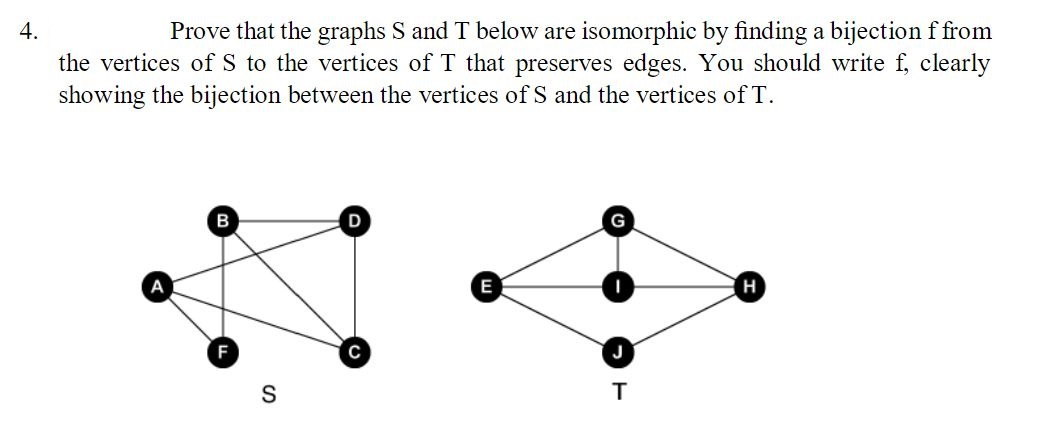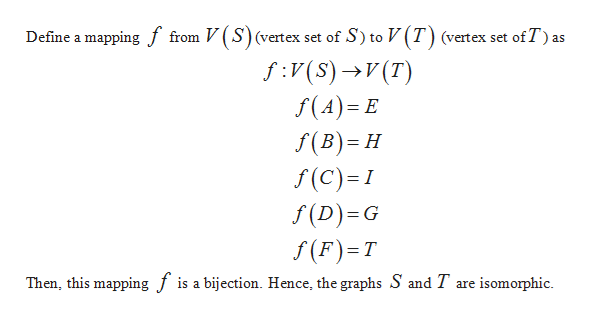# Prove that the graphs S andbelow are isomorphic by finding a bijection ffrom4.the vertices of S to the vertices of T that preserves edges. You should write f, clearlyshowing the bijection between the vertices ofS and the vertices of TBGнEстS

Question
56 views

Discrete math graph theoryhelp_outlineImage TranscriptioncloseProve that the graphs S and below are isomorphic by finding a bijection ffrom 4. the vertices of S to the vertices of T that preserves edges. You should write f, clearly showing the bijection between the vertices ofS and the vertices of T B G н E с т S fullscreen
check_circle

Step 1

Two graphs S and T are isomorphic if there exists a bijection f between the vertex sets of S and T such that an...help_outlineImage TranscriptioncloseDefine a mapping f from V (S)(vertex set of S) to V(T) (vertex set ofT) as f:V(s)V(T) f(A) E f(B) H f(C) I f (D) G f(F) T Then, this mapping f is a bijection. Hence, the graphs S and T are isomorphic fullscreen

### Want to see the full answer?

See Solution

#### Want to see this answer and more?

Solutions are written by subject experts who are available 24/7. Questions are typically answered within 1 hour.*

See Solution
*Response times may vary by subject and question.
Tagged in